# Geometrical Optics 101: Paraxial Ray Tracing Calculations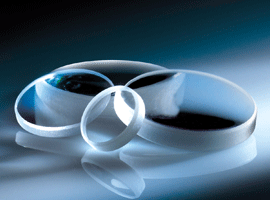Ray tracing is the primary method used by optical engineers to determine optical system performance. Ray tracing is the act of manually tracing a ray of light through a system by calculating the angle of refraction/reflection at each surface. This method is extremely useful in systems with many surfaces, where Gaussian and Newtonian imaging equations are unsuitable given the degree of complexity.

Today, ray tracing software such as ZEMAX® or CODE V® enable optical engineers to quickly simulate the performance of very complicated systems. Paraxial ray tracing involves small ray angles and heights. To understand the basic principles of paraxial ray tracing, consider the necessary calculations and ray tracing tables employed in manually tracing rays of light through a system. This will in turn highlight the usefulness of modern computing software.

## PARAXIAL RAY TRACING STEPS: CALCULATING BFL OF A PCX LENS

Paraxial ray tracing by hand is typically done with the aid of a ray-tracing sheet (Figure 1). The number of optical lens surfaces is indicated horizontally and the key lens parameters vertically. There are also sections to differentiate the marginal and chief ray. Table 1 explains the key optical lens parameters.

To illustrate the steps in paraxial ray tracing by hand, consider a plano-convex (PCX) lens. For this example, #49-849 25.4mm Diameter × 50.8mm FL lens is used for simplicity. This particular calculation is used to calculate the back focal length $\small{ \left( \text{BFL} \right)}$ of the PCX lens, but it should be noted that ray tracing can be used to calculate a wide variety of system parameters ranging from cardinal points to pupil size and location.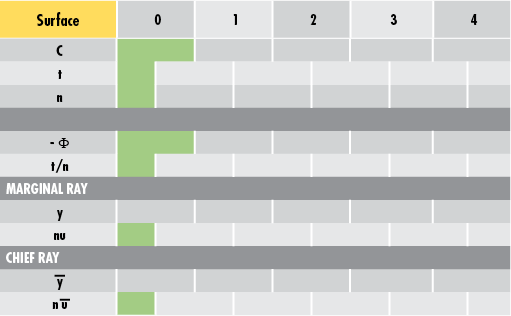### Step 1: Enter Known Values

To begin, enter the known dimensional values of #49-849 into the ray-tracing sheet (Figure 2). Surface 0 is the object plane, Surface 1 is the convex surface of the lens, Surface 2 is the plano surface of the lens, and Surface 3 is the image plane (Figure 3).

Remember that the curvature $\small{ \left( C \right)}$ is equivalent to 1 divided by the radius of curvature $\small{\left( R \right)}$. The first thickness value $\small{ \left( t \right)}$ (25mm in this example) is the distance from the object to the first surface of the lens. This value is arbitrary for incident collimated light (i.e. light parallel to the optical axis of the optical lens). The index of refraction $\small{ \left( n \right)}$ can be approximated as 1 in air and as 1.517 for the N-BK7 substrate of the lens.

VariableDescription
$$C$$ Curvature
$$t$$ Thickness
$$n$$ Index of Refraction
$$\Phi$$ Surface Power
$$y$$ Ray Height
$$u$$ Ray Angle

Table 1: Optical Lens Parameters for Ray Tracing

In Figure 2, the red box is the value to be calculated because it is the distance from the second surface to the point of focus (BFL). The power $\left( \Phi \right)$ of the individual surfaces is given by the fourth line and is calculated using Equation 1. Note: A negative sign is added to this line to make further calculations easier. In this example, Surface 1 is the only surface with power as it is the only curved surface in the system.

(1)$$\Phi = \left( n_2 - n_1 \right) C_1$$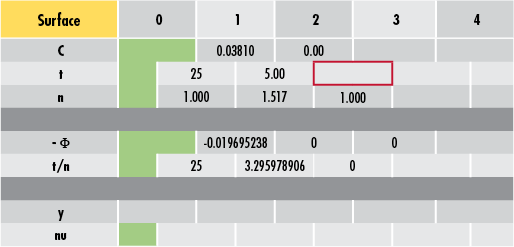##### Figure 2: Entering Known Lens Parameter Values into Ray-Tracing Sheet### Step 2: Add a Marginal Ray to the System

The next step is to add a marginal ray to the system. Since the PCX lens is spherical with a constant radius of curvature and a collimated input beam is used, the ray height $\small{\left( y \right)}$ is arbitrary. To simplify calculations, use a height of 1mm.

A collimated beam also means the initial ray angle $\small{\left( u \right)}$ is $\small{0}°$. In the ray-tracing sheet, $\small{ n \, u }$ is simply the angle of the ray multiplied by the refractive index of that medium. Both variables are included to make subsequent calculations simpler (Figure 4).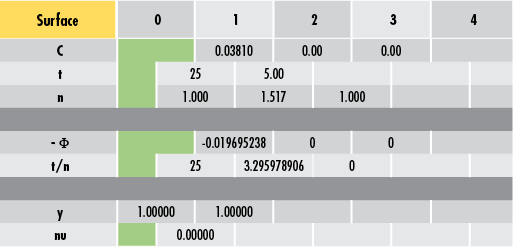### Step 3: Calculate BFL with Equations and the Ray Tracing Sheet

Ray tracing involves two primary equations in addition to the one for calculating power. Equations 2 – 3 are necessary for any ray-tracing calculations.

(2)$$y' = y + u't'$$

(3)$$n'u' = nu - y \Phi$$

where an apostrophe denotes the subsequent surface, angle, thickness, etc. In this example, to find the ray height at Surface 2 $\small{ \left( y' \right) }$, take the ray height at Surface 1 $\small{ \left( y \right) }$ and add it to -0.0197 multiplied by 3.296:

(2.1)\begin{align} y' & = y + u't' \\ & = y + \left( \frac{t'}{n'} \right) n'u' \\ & = 1 + \left( 3.296 \right) \left( -0.0197 \right) = 0.93508 \end{align}

Performing this for ray angle yields the following value. The entire process is repeated until the ray trace is complete (Figure 5).

(3.1)\begin{align} n'u' & = nu - y \Phi \\ & = -0.0197 + \left( 0.93508 \right) \left( -0 \right) = -0.0197 \end{align}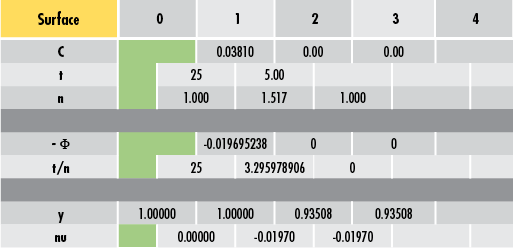##### Figure 5: Propagating the Ray through the System

Now, solve for the $\small{\text{BFL}}$ by either adjusting the thickness value until the final ray height is 0 (Figure 6) or by backward calculating the $\small{\text{BFL}}$ for a ray height of 0. For #49-849, the final $\small{\text{BFL}}$ value is 47.48mm. This is very close to the 47.50mm listed in the lens' specifications. The difference is attributed to the rounding error of using an index of refraction of 1.517 instead of a slightly more accurate value that was used when the lens was initially designed.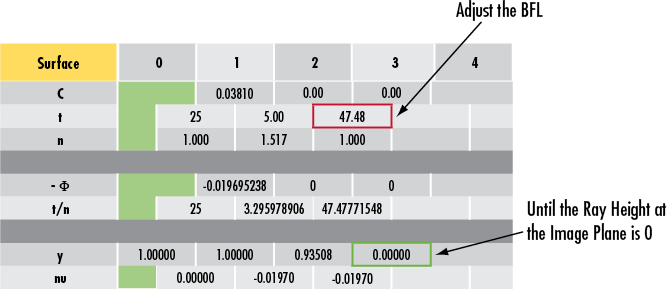## DECIPHERING A TWO-LENS RAY TRACING SHEET

To completely understand a ray tracing sheet, consider a two-lens system consisting of a double-concave (DCV) lens, an iris, and a double-convex (DCX) lens (Figures 7 - 8). To learn more about DCV and DCX lenses, please read Understanding Optical Lens Geometries.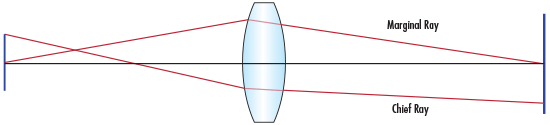##### Figure 7: Double-Concave (DCV) and Double-Convex (DCX) Lens System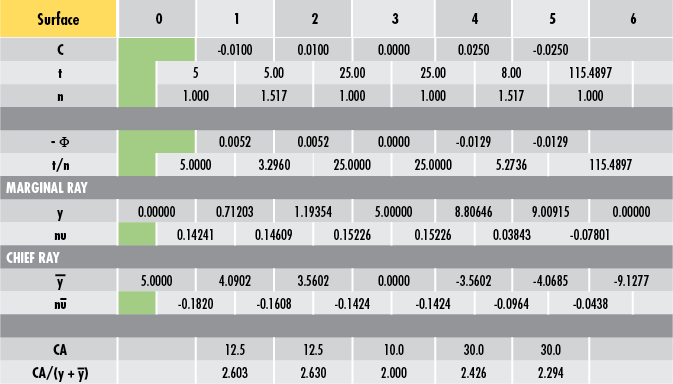##### Figure 8: Sample Double-Concave (DCV) and Double-Convex (DCX) Ray Tracing System

The aperture stop is the limiting aperture and defines how much light is allowed through the system. The aperture stop can be an optical lens surface or an iris, but it is always a physical surface. The entrance pupil is the image of the aperture stop when it is imaged through the preceding lens elements into object space. The exit pupil is the image of the aperture stop when it is imaged through the following lens elements into image space.

In an optical system, the aperture stop and the pupils are used to define two very important rays. The chief ray is one that begins at the edge of the object and goes through the center of the entrance pupil, exit pupil, and the stop (in other words, it has a height $\small{\left( \bar{y} \right)}$ of 0 at those locations). The chief ray, therefore, defines the size of the object and image and the locations of the pupils.

The marginal ray of an optical system begins on-axis at the object plane. This ray encounters the edge of the pupils and stops and crosses the axis at the object and image points. The marginal ray, therefore, defines the location of the object and image and the sizes of the pupils.

### Aperture Stop Location

If the location of the aperture stop is unknown, a trial ray, known as the pseudo marginal ray, must be propagated through the system. For an object not at infinity, this ray must begin at the axial position of the object and can have an arbitrary incident angle. For an object at infinity, the ray can begin at an arbitrary height, but must have an incident angle of $\small{0} °$. Once this is accomplished, the aperture stop is simply the surface that has the smallest $\tfrac{\text{CA}}{y_p}$ (also stylized as $\small{\text{CA}/y_p}$ ) value, where $\small{\text{CA}}$ is the surface clear aperture and $\small{y_p}$ is the height of the pseudo marginal ray at that surface.

After locating the aperture stop, the pseudo marginal ray can be scaled appropriately to obtain the actual marginal ray (remember the marginal ray should touch the edge of the aperture stop). Once the size and location of the aperture stop are known, the marginal ray height is equal to the radius of the stop and the chief ray height is zero at that location. Paraxial ray tracing can then be carried out in both the forward and the reverse directions from those points. When doing ray tracing in reverse, Equations 4 – 5 are useful. Note the similarities to Equations 2 – 3.

(4)$$y = y' - u't'$$

(5)$$nu = n'u' + y \Phi$$

### Vignetting Analysis

Once the location and size of the aperture stop are known, use vignetting analysis to see which surfaces will vignette, or cause rays to be blocked. Vignetting analysis is accomplished by taking the clear aperture at every surface and dividing it by two. That value is then compared to the heights of the chief and marginal rays at that surface (Equation 6). Equation 6 can be easily reordered to Equation 7. If Equation 7 is true, the surface does not vignette.

(6)$$\frac{\text{CA}}{2} \geq \left| \bar{y} \right| + \left| y \right|$$

(7)$$\frac{\text{CA}}{\left| \bar{y} \right| + \left| y \right|} \geq 2$$

Notice in the preceding DCV and DCX example how Surface 3 is the aperture stop where the $\tfrac{\text{CA}}{\left( \left| \bar{y} \right| + \left| y \right| \right)}$ value is the smallest among all surfaces. Also, none of the surfaces vignette because all values are greater than or equal to 2.

### Object/Image Size and Location

Object (Surface 0)

• Size is 10mm in diameter (twice the chief ray height at Surface 0)
• Location is 5mm in front of the first lens (the first thickness value)

Image (Surface 6)

• Size is 18.2554mm in diameter (twice the final chief ray height)
• Location is 115.4897mm behind the final lens surface (the last thickness value)

It is important to note that the Surface 0 chief ray height is positive while the Surface 6 chief ray height is negative. This indicates that the image is inverted.

### Effective Focal Length

To solve for the effective focal length $\small{ \left( \text{EFL} \right)}$, it is first necessary to trace a pseudo marginal ray through the system for an object at infinity (i.e. the first ray angle will be 0). In Figure 9, an arbitrary initial height of 1 is chosen to simplify calculations. Once this is accomplished, the $\small{ \text{EFL}}$ of the system is given by Equation 8.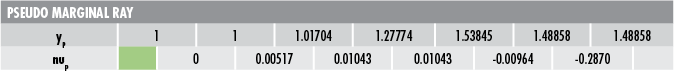##### Figure 9: Pseudo Marginal Ray

(8)\begin{align} \text{EFL} & = \frac{y_1}{nu_{\text{last}}} \\ & = \frac{1}{0.02870} = 34.843 \text{mm} \end{align}

(9)\begin{align} \text{FOV} & = 2 \left( n \bar{u} \right) \\ & = 2 \left( 0.182 \right) = 0.364 \text{radians} = 20.856° \end{align}

where $\small{n \, \bar{u}}$ is the first chief ray angle.

### Lagrange Invariant

The optical invariant is a useful tool that allows optical designers to determine various values without having to completely ray trace a system. It is obtained by comparing two rays within a system at any axial point. The optical invariant is constant for any two rays at every point in the system. In other words, if the invariant for a set of two rays is known, ray trace one of the rays and then scale that by the invariant to find the second.

The Lagrange Invariant is a version of the optical invariant that uses the chief ray and the marginal ray as the two rays of interest. It is solved using Equation 10 and is illustrated in Figure 10.

(10)$$Ж = n \bar{u} y - n u \bar{y}$$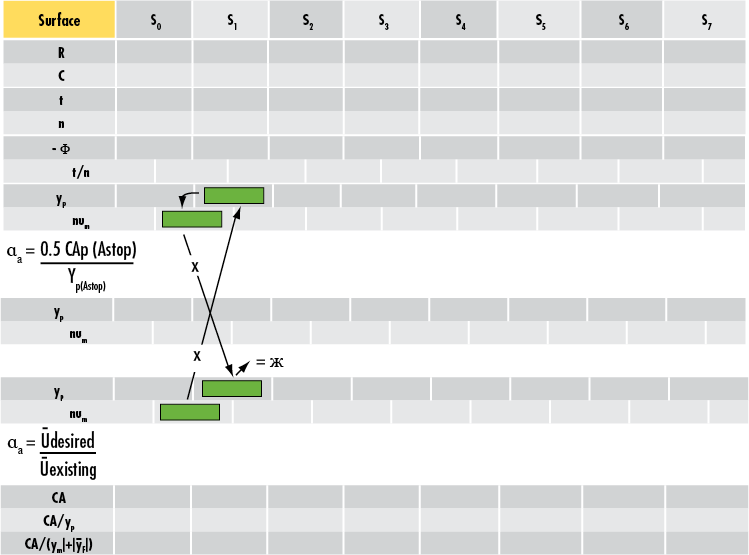## REAL-WORLD RAY TRACING AND SOFTWARE ADVANTAGES

Within paraxial ray tracing, there are several assumptions that introduce error into the calculations. Paraxial ray tracing assumes that the tangent and sine of all angles are equal to the angles themselves (in other words, $\small{\tan{\left( u \right)} = u}$ and $\small{\sin{\left( u \right)} = u}$). This approximation is valid for small angles but can lead to the propagation of error as ray angles increase.

Real ray tracing is a method of reducing paraxial error by eliminating the small-angle approximation and by accounting for the sag of each surface to better model the refraction of off-axis rays. As with paraxial ray tracing, real ray tracing can be done by hand with the help of a ray trace sheet. For the sake of brevity, only the paraxial method has been demonstrated. Ray tracing software such as CODE V and ZEMAX use real ray tracing to model user-inputted optical systems.

Ray tracing by hand is a tedious process. Consequently, ray tracing software is usually the preferred method of analysis. Figure 11 shows the DCV-DCX system from the section on "Deciphering a Two Lens Ray Tracing Sheet". The following ZEMAX screenshot shows a focal length value of 34.699mm – confirming the paraxial calculation previously performed.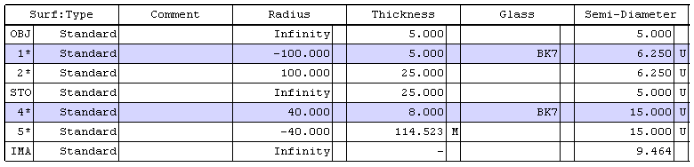##### Figure 11: Sample ZEMAX System Data

Ray tracing is an important tool for any optical designer. While the proliferation of ray tracing software has minimized the need for paraxial ray tracing by hand, it is still useful to understand conceptually how individual rays of light move through an optical system. Paraxial ray tracing and real ray tracing are great ways to approximate optical lens performance before finalizing a design and going into production. Without ray tracing, system design is much more difficult, expensive, and time-intensive.

### References

1. Dereniak, Eustace L., and Teresa D. Dereniak. "Chapter 10. Paraxial Ray Tracing." In Geometrical and Trigonometric Optics, 255-91. Cambridge, UK: Cambridge University Press, 2008.
2. Geary, Joseph M. "Chapter 4 – Paraxial World." In Introduction to Lens Design: With Practical Zemax Examples, 33-42. Richmond, VA: Willmann-Bell, 2002.
3. Greivenkamp, John E. "Paraxial Raytrace." In Field Guide to Geometrical Optics, 20-32. Vol. FG01. Bellingham, WA: SPIE—The International Society for Optical Engineers, 2004.
4. Smith, Warren J. "Chapter 3. Paraxial Optics and Calculations." In Modern Optical Engineering, 35-51. 4th ed. New York, NY: McGraw-Hill Education, 2007.

$\small{y}$